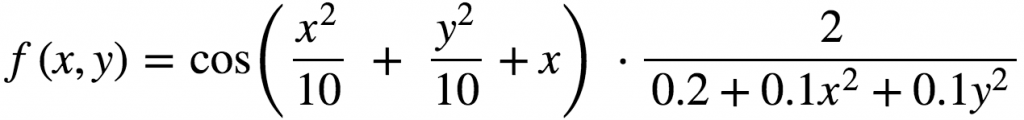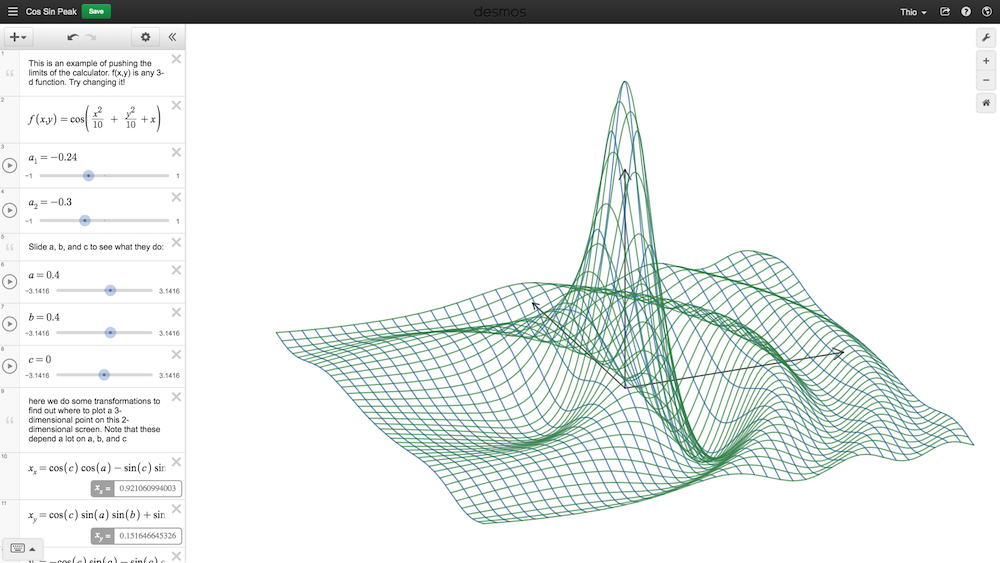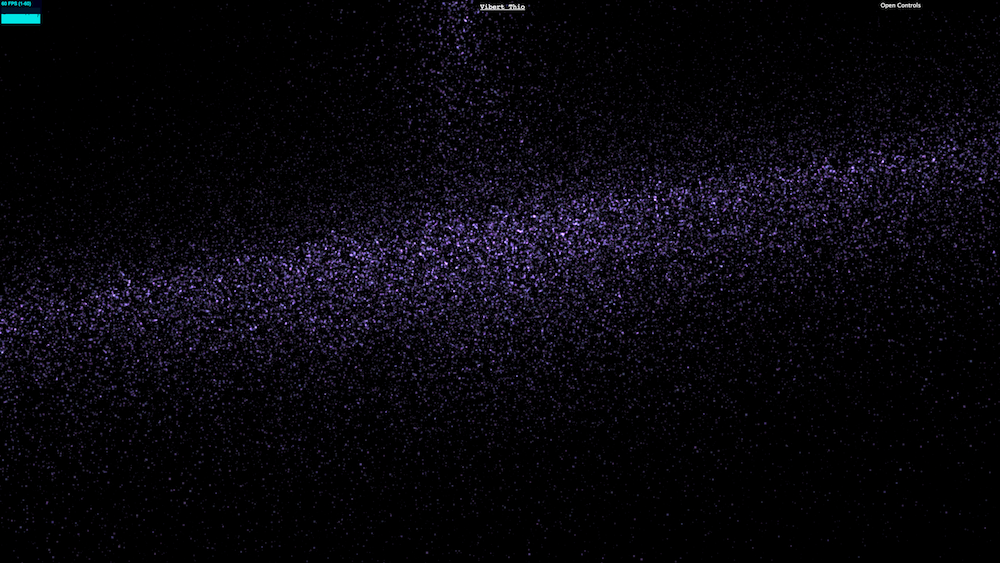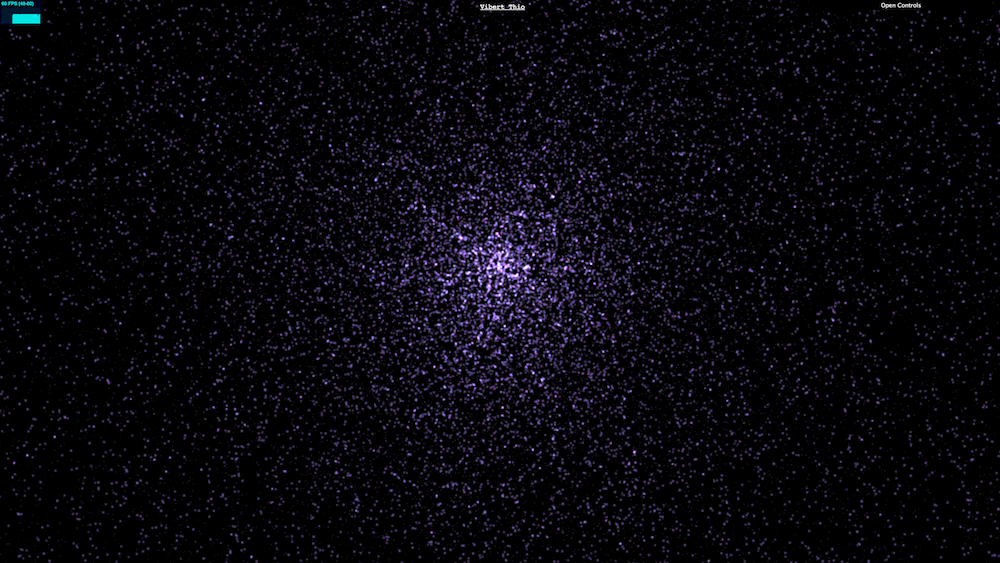#2018 iT 邦幫忙鐵人賽DAY 2
2
Modern Web

## §ｄ０２§ 錐細胞衝擊！時空扭曲！數學模擬黑洞造型。## 0. 今日工事

• 重力塌陷時空扭曲
• 來吧！畫出來吧！

### 上集提要

§ｄ１§ 錐細胞衝擊！放射系宇宙！用 Three.js 的 particle system 當創世神！

``````// preload random
let rand = [];
let randIndex;
for (randIndex = 1e5; randIndex > 0; randIndex -= 1) {
rand.push(Math.random());
}
function random() {
return ++ randIndex >= rand.length ? rand[ randIndex = 1 ] : rand[ randIndex ];
}
``````

## 2. 重力塌陷時空扭曲## 3. 來吧！畫出來吧！

``````function newShape() {
const r = 40;
const x = r * (random() - 0.5);
const y = r * (random() - 0.5);
const x2 = x * x;
const y2 = y * y;
const z = Math.cos(x2 * 0.1 + y2 * 0.1 + x) * 2 / (0.2 + 0.1 * x2 + 0.1 * y2);

return ({
x,
y,
z,
});
}
````````````// 這邊的 rate 是控制集中效果的參數，高高
function newShape(rate) {
const r = 100;
const angle = random() * Math.PI * 2;
const rr = random();
const radius = Math.pow(rr, rate) * r;

const x = radius * Math.cos(angle);
const y = radius * Math.sin(angle);
const x2 = x * x;
const y2 = y * y;
const z = Math.cos(x2 * 0.1 + y2 * 0.1 + x) * 2 / (0.2 + 0.1 * x2 + 0.1 * y2);

return ({
x,
y: z,
z: y,
});
}
``````## 4. 請愛ＣＹＢＥＲの ａｕｄｉｏ / ＶＩＳＵＡＬ

To be or not to be? 明天該是來研究為什麼可以用 three 這種黑魔法來畫圖的時候了。應該會研究 shader，還有裡面使用的 buffer, attribute 等。可怕啊，可怕啊。